# Bookshelf and books

How many ways can we place 7 books in a bookshelf?

Correct result:

n =  5040

#### Solution:

$n=7!=5040$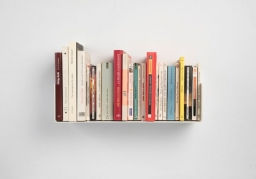We would be pleased if you find an error in the word problem, spelling mistakes, or inaccuracies and send it to us. Thank you!

Showing 1 comment:Math student
Needs rephrasing >>Tips to related online calculators
Would you like to compute count of combinations?

## Next similar math problems:

• VariationsDetermine the number of items when the count of variations of fourth class without repeating is 42 times larger than the count of variations of third class without repetition.
• Big factorial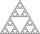How many zeros end number 116! ?
• PIN - codesHow many five-digit PIN - code can we create using the even numbers?
• Olympics metalsIn how many ways can be win six athletes medal positions in the Olympics? Metal color matters.
• Elections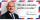In elections candidate 10 political parties. Calculate how many possible ways can the elections finish, if any two parties will not get the same number of votes.
• MedalsIn how many ways can be divided gold, silver and bronze medal among 21 contestant?
• Football league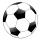In the 5th football league is 10 teams. How many ways can be filled first, second and third place?
• DigitsHow many natural numbers greater than 4000 which are formed from the numbers 0,1,3,7,9 with the figures not repeated, B) How many will the number of natural numbers less than 4000 and the numbers can be repeated?
• Vans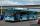In how many ways can 9 shuttle vans line up at the airport?
• Research in schoolFor particular research in high school, four pupils are to be selected from a class with 30 pupils. Calculate the number of all possible results of the select and further calculate the number of all possible results, if it depends on the order in which th
• Three digits numberHow many are three-digit integers such that in they no digit repeats?
• Guests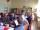How many ways can 7 guests sit down on 8 seats standing in a row?
• DigitsHow many five-digit numbers can be written from numbers 0.3,4, 5, 7 that is divided by 10 and if digits can be repeated.
• Pairs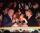At the table sit 10 people, 5 on one side and 5 on the other side. Among them are 3 pairs. Every pair wants to sit opposite each other. How many ways can they sit?
• Seating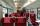How many ways can 6 people sit on 6 numbered chairs (e. G. , seat reservation on the train)?
• Practice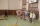How many ways can you place 20 pupils in a row when starting on practice?
• Boys and girlsThere are 20 boys and 10 girls in the class. How many different dance pairs can we make of them?